Type
Quiz
Book Title
Fundamentals of Corporate Finance Standard Edition 9th Edition
ISBN 13
978-0073382395

### 978-0073382395 Chapter 14 Questions and Problems 1-20

April 3, 2019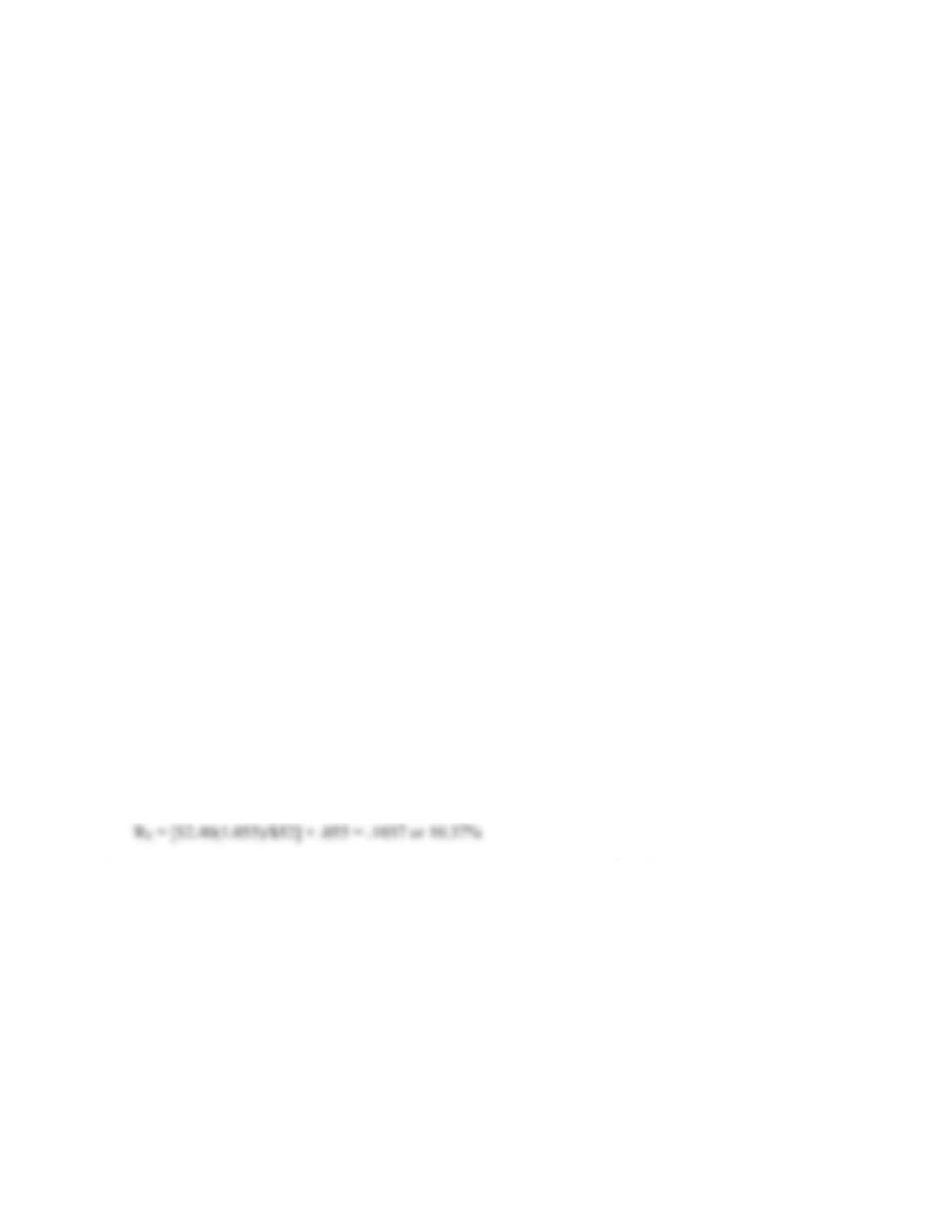B-252 SOLUTIONS
8. a. This only considers the dividend yield component of the required return on equity.
b. This is the current yield only, not the promised yield to maturity. In addition, it is based on the
book value of the liability, and it ignores taxes.
c. Equity is inherently more risky than debt (except, perhaps, in the unusual case where a firm’s
assets have a negative beta). For this reason, the cost of equity exceeds the cost of debt. If taxes are
considered in this case, it can be seen that at reasonable tax rates, the cost of equity does exceed
the cost of debt.
9. R
Sup = .12 + .75(.08) = .1800 or 18.00%
Both should proceed. The appropriate discount rate does not depend on which company is investing; it
depends on the risk of the project. Since Superior is in the business, it is closer to a pure play. Therefore,
its cost of capital should be used. With an 18% cost of capital, the project has an NPV of \$1 million
regardless of who takes it.
10. If the different operating divisions were in much different risk classes, then separate cost of capital
figures should be used for the different divisions; the use of a single, overall cost of capital would be
inappropriate. If the single hurdle rate were used, riskier divisions would tend to receive more funds for
investment projects, since their return would exceed the hurdle rate despite the fact that they may
actually plot below the SML and, hence, be unprofitable projects on a risk-adjusted basis. The typical
problem encountered in estimating the cost of capital for a division is that it rarely has its own securities
traded on the market, so it is difficult to observe the market’s valuation of the risk of the division. Two
typical ways around this are to use a pure play proxy for the division, or to use subjective adjustments of
the overall firm hurdle rate based on the perceived risk of the division.
Solutions to Questions and Problems
NOTE: All end of chapter problems were solved using a spreadsheet. Many problems require multiple steps.
Due to space and readability constraints, when these intermediate steps are included in this solutions
manual, rounding may appear to have occurred. However, the final answer for each problem is found
without rounding during any step in the problem.
Basic
1. With the information given, we can find the cost of equity using the dividend growth model. Using this
model, the cost of equity is:
2. Here we have information to calculate the cost of equity using the CAPM. The cost of equity is:
RE = .053 + 1.05(.12 – .053) = .1234 or 12.34%
3. We have the information available to calculate the cost of equity using the CAPM and the dividend
growth model. Using the CAPM, we find:
RE = .05 + 0.85(.08) = .1180 or 11.80%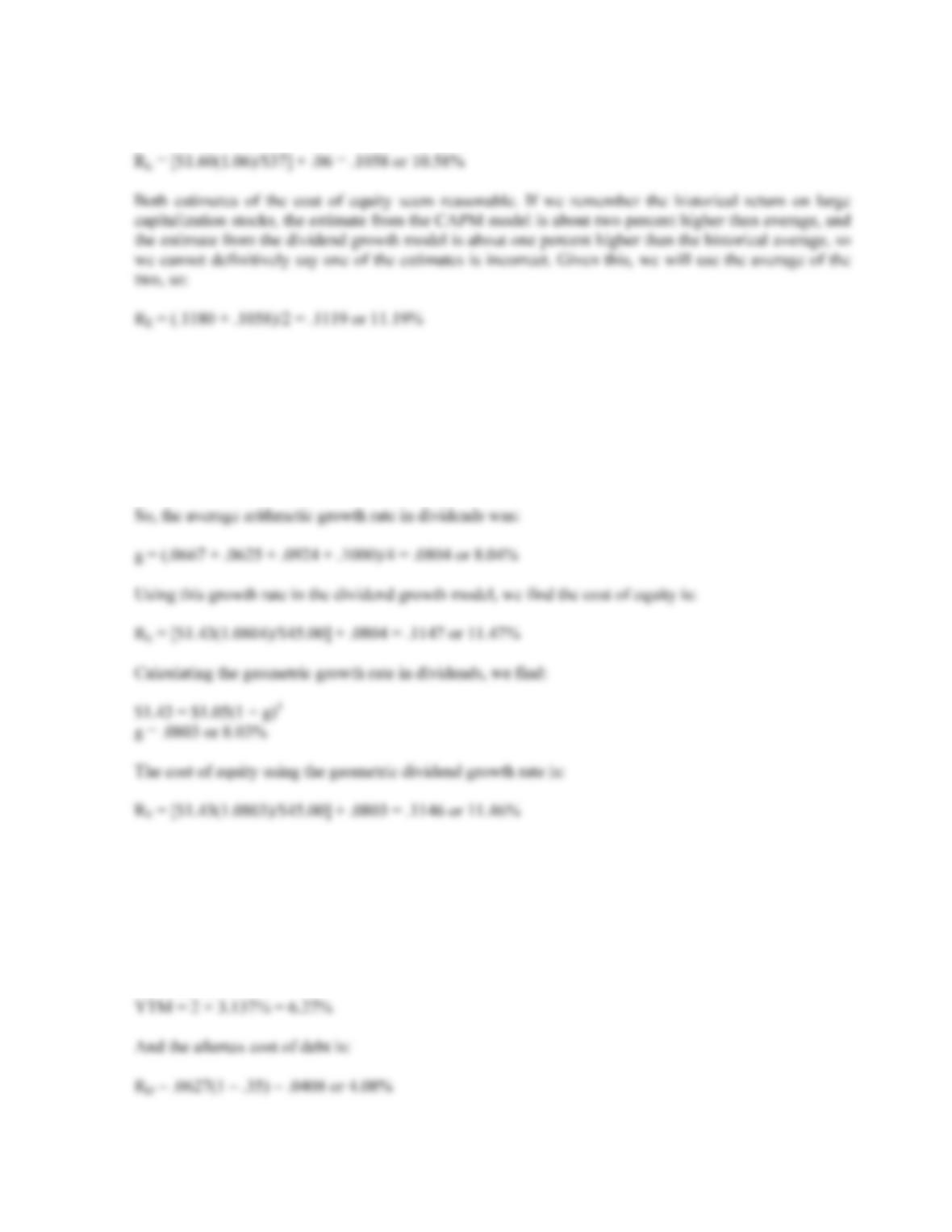CHAPTER 14 B-253
And using the dividend growth model, the cost of equity is
4. To use the dividend growth model, we first need to find the growth rate in dividends. So, the increase in
dividends each year was:
g1 = (\$1.12 – 1.05)/\$1.05 = .0667 or 6.67%
g
2 = (\$1.19 – 1.12)/\$1.12 = .0625 or 6.25%
g3 = (\$1.30 – 1.19)/\$1.19 = .0924 or 9.24%
g
4 = (\$1.43 – 1.30)/\$1.30 = .1000 or 10.00%
5. The cost of preferred stock is the dividend payment divided by the price, so:
RP = \$6/\$96 = .0625 or 6.25%
6. The pretax cost of debt is the YTM of the company’s bonds, so:
P0 = \$1,070 = \$35(PVIFAR%,30) + \$1,000(PVIFR%,30)
R = 3.137%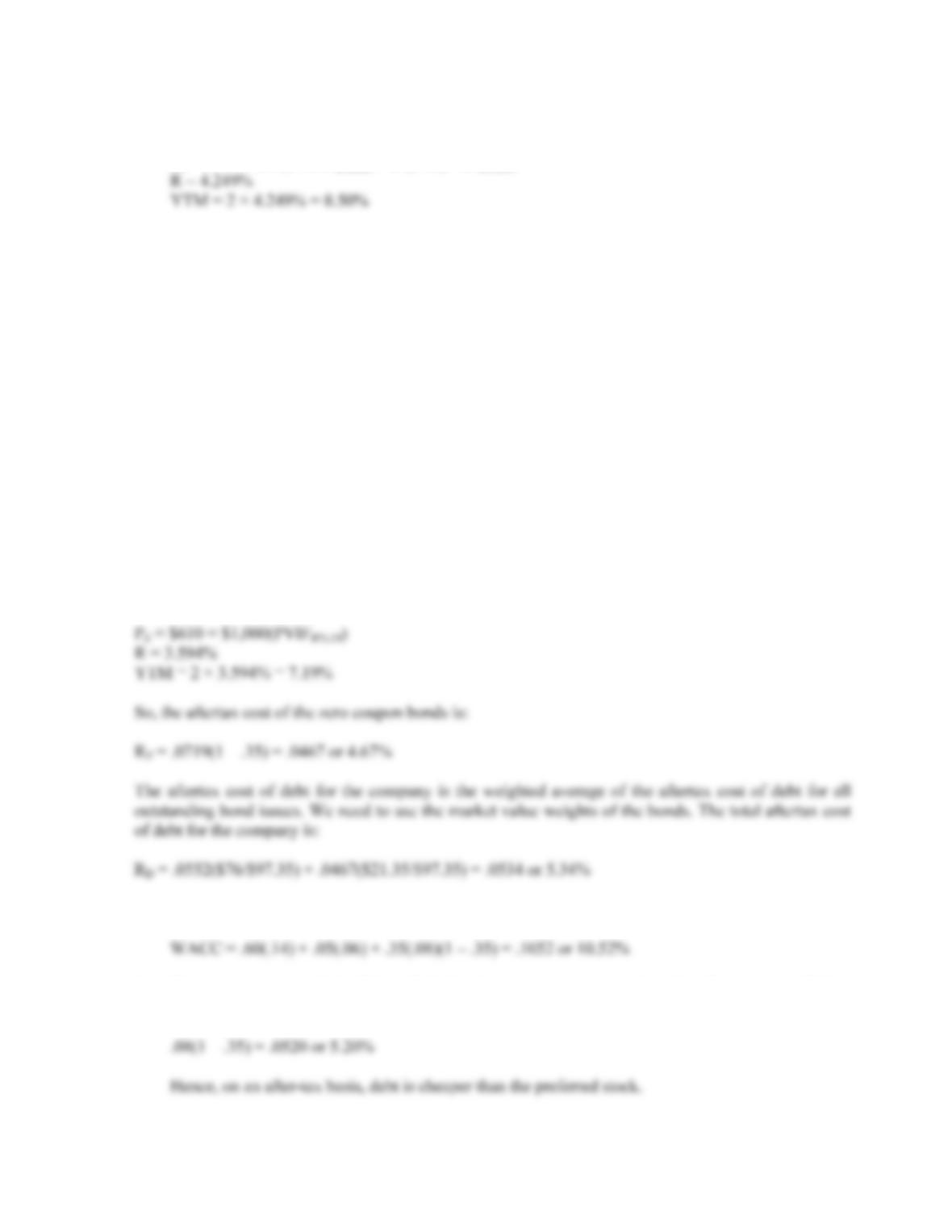B-254 SOLUTIONS
7. a. The pretax cost of debt is the YTM of the company’s bonds, so:
P
0 = \$950 = \$40(PVIFAR%,46) + \$1,000(PVIFR%,46)
b. The aftertax cost of debt is:
R
D = .0850(1 – .35) = .0552 or 5.52%
c. The after-tax rate is more relevant because that is the actual cost to the company.
8. The book value of debt is the total par value of all outstanding debt, so:
BVD = \$80,000,000 + 35,000,000 = \$115,000,000
To find the market value of debt, we find the price of the bonds and multiply by the number of bonds.
Alternatively, we can multiply the price quote of the bond times the par value of the bonds. Doing so,
we find:
MVD = .95(\$80,000,000) + .61(\$35,000,000)
MVD = \$76,000,000 + 21,350,000
MVD = \$97,350,000
The YTM of the zero coupon bonds is:
9. a. Using the equation to calculate the WACC, we find:
b. Since interest is tax deductible and dividends are not, we must look at the after-tax cost of debt,
which is: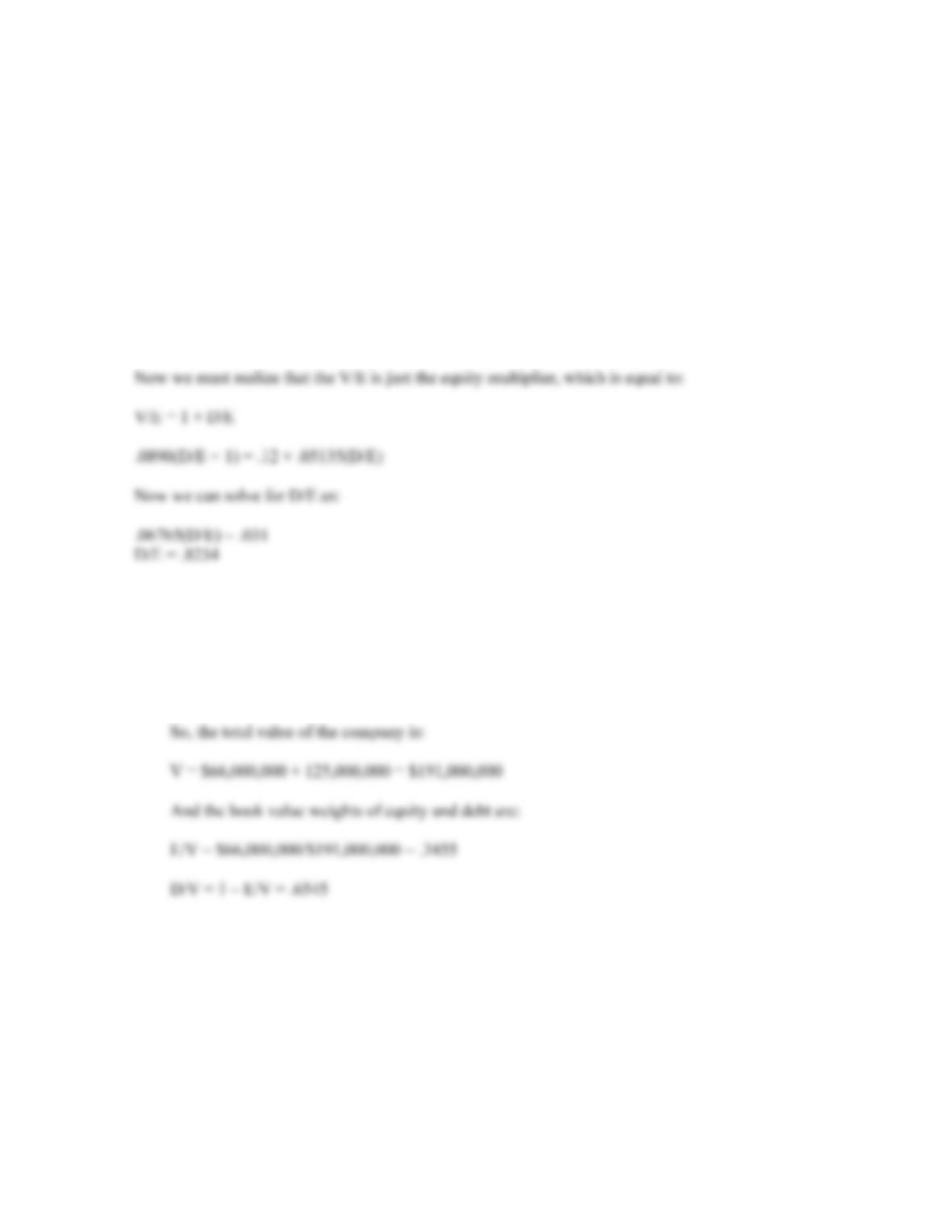CHAPTER 14 B-255
10. Here we need to use the debt-equity ratio to calculate the WACC. Doing so, we find:
WACC = .15(1/1.65) + .09(.65/1.65)(1 – .35) = .1140 or 11.40%
11. Here we have the WACC and need to find the debt-equity ratio of the company. Setting up the WACC
equation, we find:
WACC = .0890 = .12(E/V) + .079(D/V)(1 – .35)
Rearranging the equation, we find:
.0890(V/E) = .12 + .079(.65)(D/E)
12. a. The book value of equity is the book value per share times the number of shares, and the book
value of debt is the face value of the company’s debt, so:
BVE = 11,000,000(\$6) = \$66,000,000
BVD = \$70,000,000 + 55,000,000 = \$125,000,000
b. The market value of equity is the share price times the number of shares, so:
MVE = 11,000,000(\$68) = \$748,000,000
Using the relationship that the total market value of debt is the price quote times the par value of
the bond, we find the market value of debt is:
MVD = .93(\$70,000,000) + 1.04(\$55,000,000) = \$122,300,000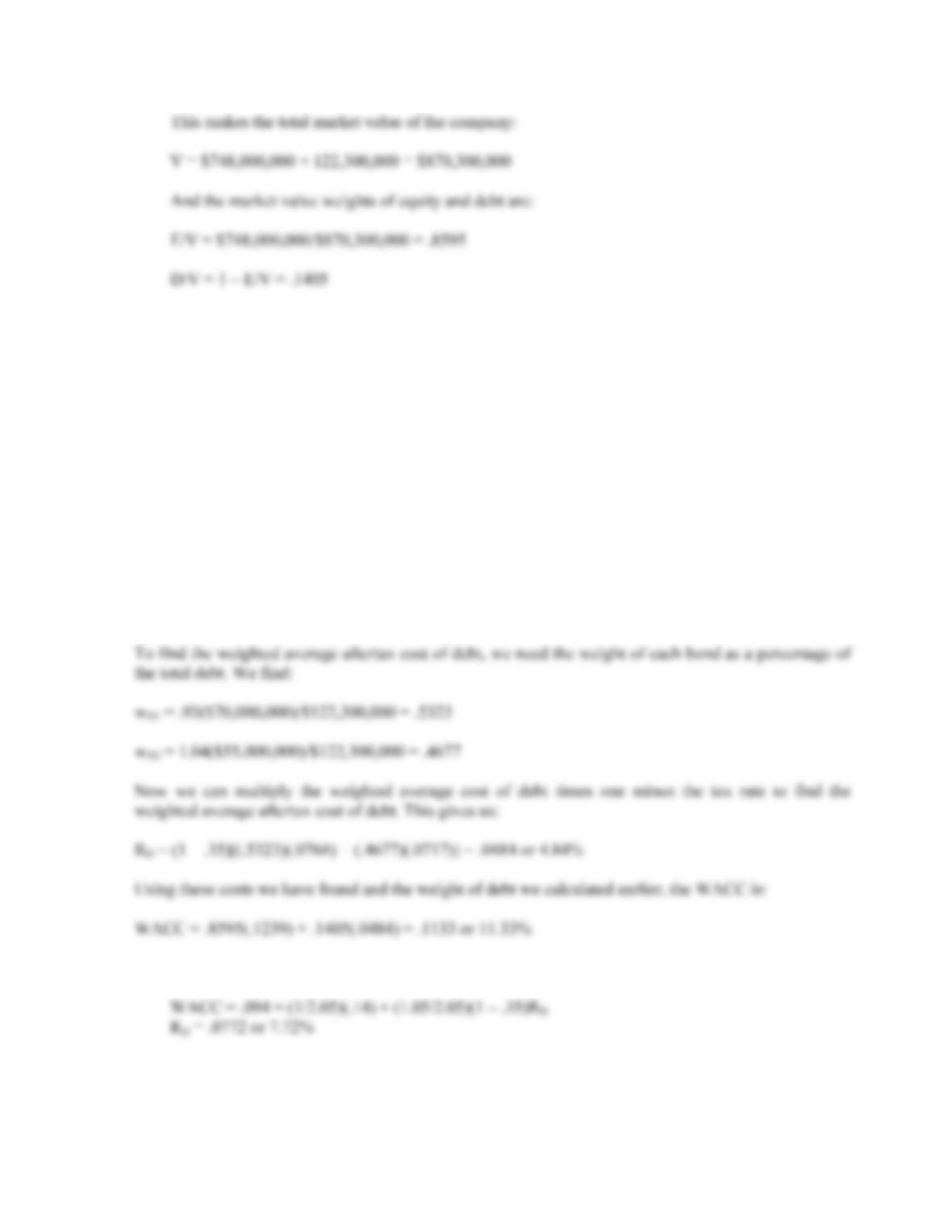B-256 SOLUTIONS
c. The market value weights are more relevant.
13. First, we will find the cost of equity for the company. The information provided allows us to solve for
the cost of equity using the dividend growth model, so:
R
E = [\$4.10(1.06)/\$68] + .06 = .1239 or 12.39%
Next, we need to find the YTM on both bond issues. Doing so, we find:
P
1 = \$930 = \$35(PVIFAR%,42) + \$1,000(PVIFR%,42)
R = 3.838%
YTM = 3.838% × 2 = 7.68%
P
2 = \$1,040 = \$40(PVIFAR%,12) + \$1,000(PVIFR%,12)
R = 3.584%
YTM = 3.584% × 2 = 7.17%
14. a. Using the equation to calculate WACC, we find: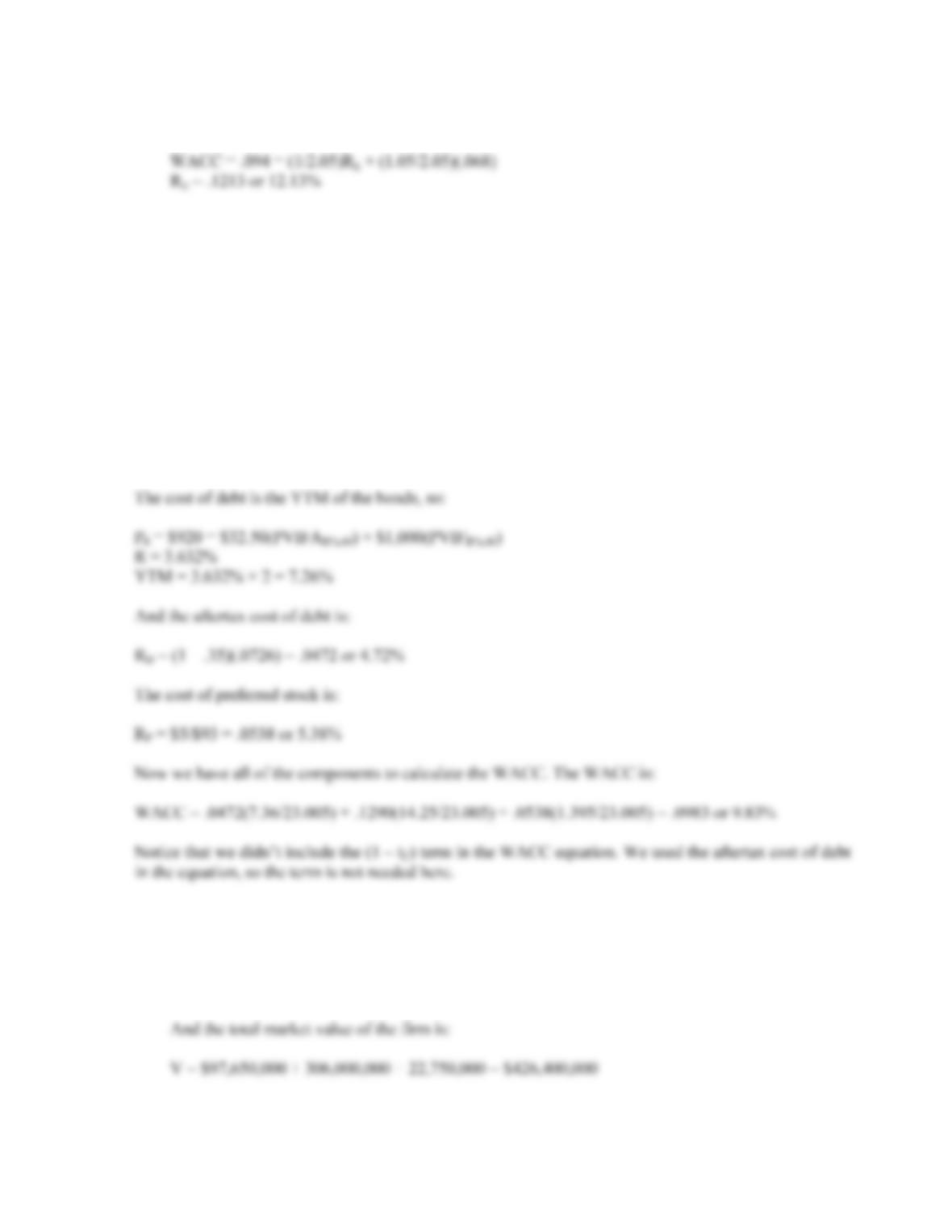CHAPTER 14 B-257
b. Using the equation to calculate WACC, we find:
15. We will begin by finding the market value of each type of financing. We find:
MVD = 8,000(\$1,000)(0.92) = \$7,360,000
MVE = 250,000(\$57) = \$14,250,000
MVP = 15,000(\$93) = \$1,395,000
And the total market value of the firm is:
V = \$7,360,000 + 14,250,000 + 1,395,000 = \$23,005,000
Now, we can find the cost of equity using the CAPM. The cost of equity is:
R
E = .045 + 1.05(.08) = .1290 or 12.90%
16. a. We will begin by finding the market value of each type of financing. We find:
MVD = 105,000(\$1,000)(0.93) = \$97,650,000
MVE = 9,000,000(\$34) = \$306,000,000
MVP = 250,000(\$91) = \$22,750,000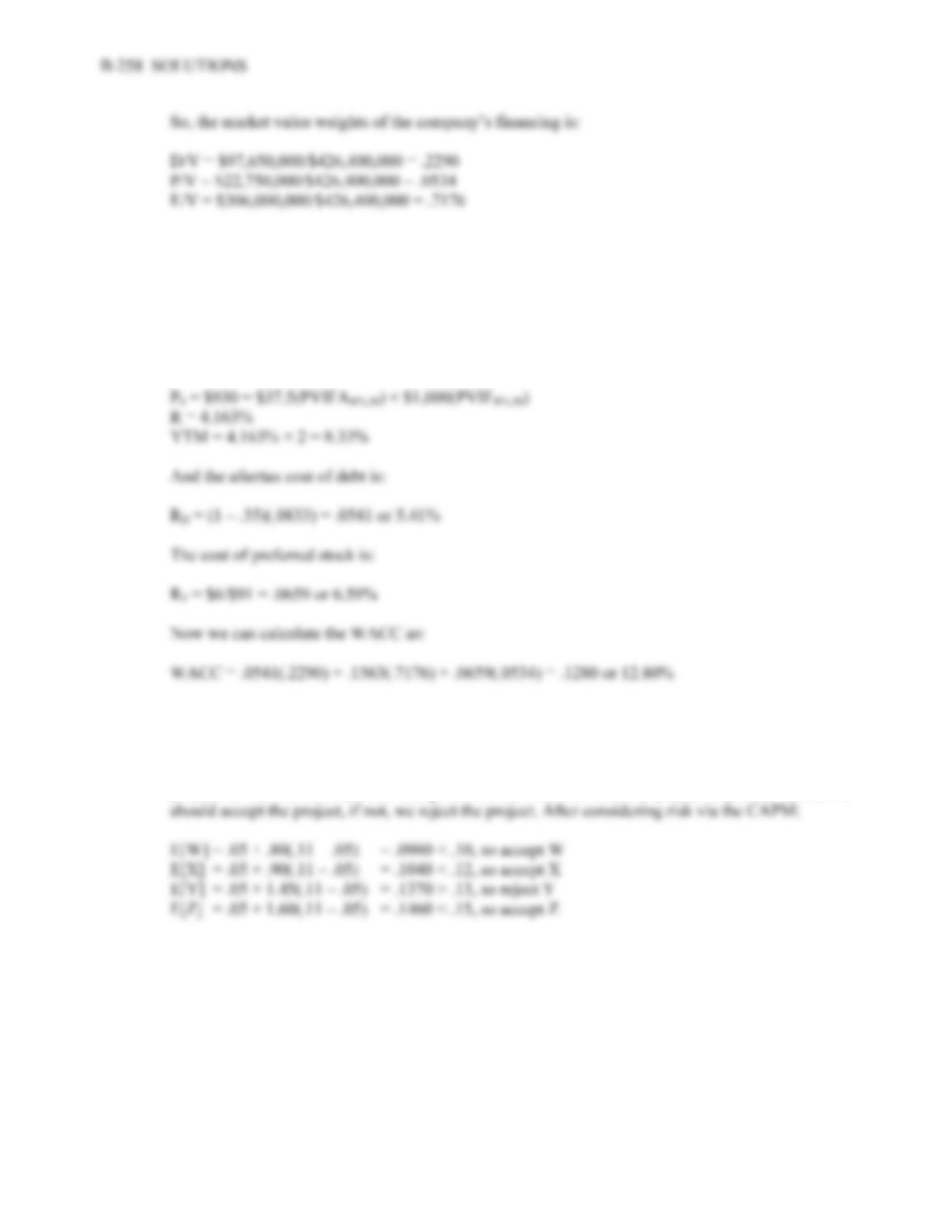b. For projects equally as risky as the firm itself, the WACC should be used as the discount rate.
First we can find the cost of equity using the CAPM. The cost of equity is:
R
E = .05 + 1.25(.085) = .1563 or 15.63%
The cost of debt is the YTM of the bonds, so:
17. a. Projects X, Y and Z.
b. Using the CAPM to consider the projects, we need to calculate the expected return of the project
given its level of risk. This expected return should then be compared to the expected return of the
project. If the return calculated using the CAPM is lower than the project expected return, we
c. Project W would be incorrectly rejected; Project Y would be incorrectly accepted.
18. a. He should look at the weighted average flotation cost, not just the debt cost.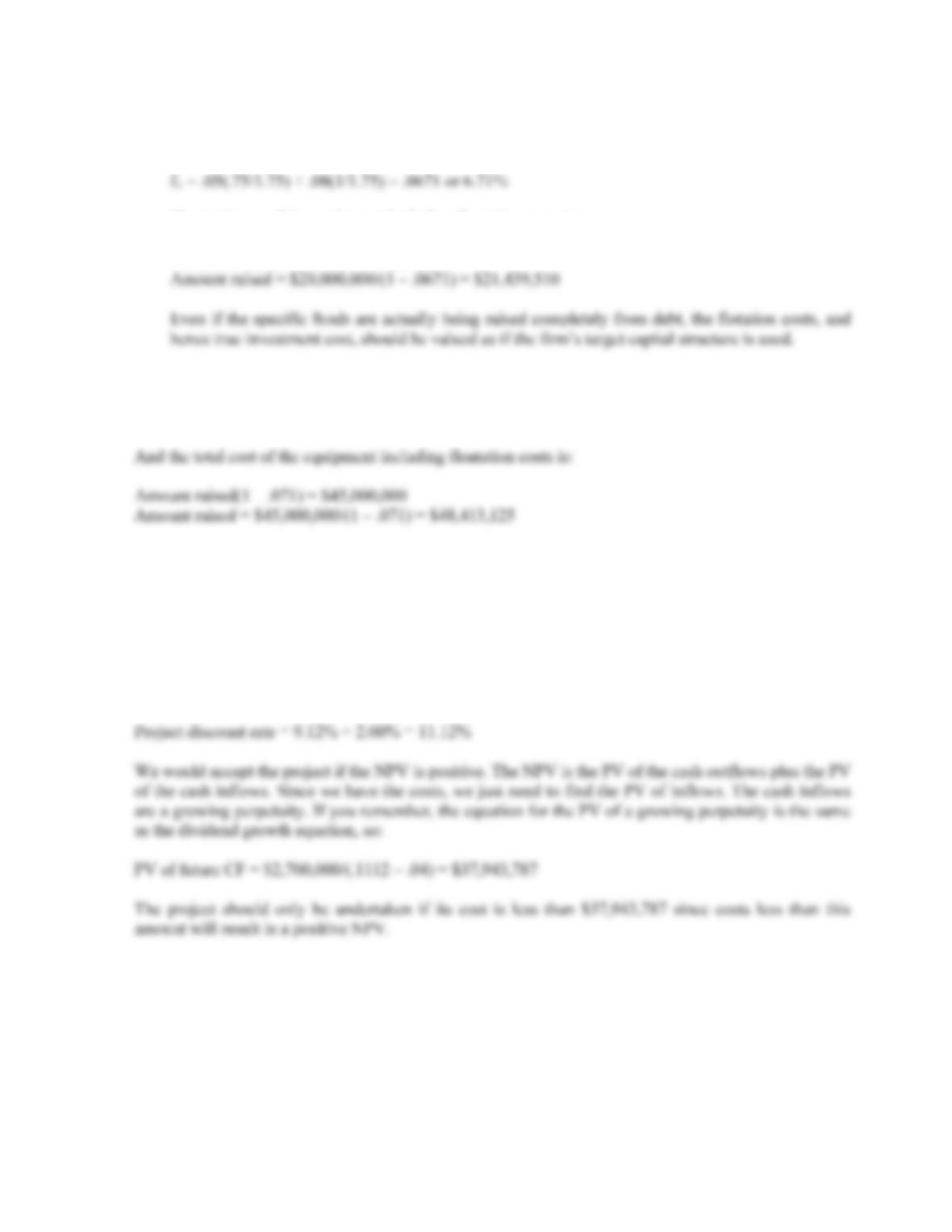CHAPTER 14 B-259
b. The weighted average floatation cost is the weighted average of the floatation costs for debt and
equity, so:
f
c. The total cost of the equipment including floatation costs is:
Amount raised(1 – .0671) = \$20,000,000
19. We first need to find the weighted average floatation cost. Doing so, we find:
f
T = .65(.09) + .05(.06) + .30(.03) = .071 or 7.1%
Intermediate
20. Using the debt-equity ratio to calculate the WACC, we find:
WACC = (.90/1.90)(.048) + (1/1.90)(.13) = .0912 or 9.12%
Since the project is riskier than the company, we need to adjust the project discount rate for the
additional risk. Using the subjective risk factor given, we find:
21. The total cost of the equipment including floatation costs was:
Total costs = \$15,000,000 + 850,000 = \$15,850,000